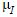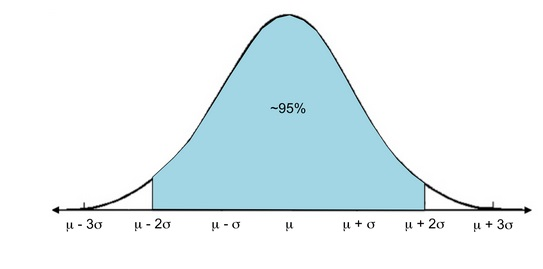PURCHASE A DIGITAL COPY PURCHASE A HARD COPY Lesson 1 Introduction to Statistical Research Methods Lesson 2 Visualizing Data Lesson 3 Central Tendency Lesson 4 Variability Lesson 5 Standardizing Lesson 6 Normal Distribution Lesson 7 Sampling Distributions Lesson 8 Estimation Lesson 9 Hypothesis Testing Lesson 10 t-Tests for Dependent Samples Lesson 11 t-Tests for Independent Samples Lesson 12 Intro to One-Way ANOVA Lesson 13 One-Way ANOVA: Test significance of differences Lesson 14 Correlation Lesson 15 Linear Regression Lesson 16 Chi-Squared Tests Afterward Index

Let’s say we apply some kind of intervention to a sample, and then find that the mean of this sample is(“I” for “intervention”). Could we use this to estimate the population parameters if everyone were to receive the same intervention?

We could guess that the new population mean, which we’ll call, would be somewhere around. From the limited information we have, the sample mean is our best estimate for the new population mean. We call this a point estimate since it’s a single value rather than a range of values.

Actually, a range of values is exactly what we want. In this lesson, we’ll calculate confidence intervals for wheremight be; in other words, we’ll be fairly confident thatis between two particular values. We’ll determine what these values should be.

In Lesson 6 you learned that for a normal distribution, most values (about 95%) are within two standard deviations of the mean.We can extend this concept to sampling distributions: approximately 95% of sample means will fall withinthe population mean.To continue learning, purchase Street-Smart Stats: A Friendly Introduction to Statistical Research Methods.